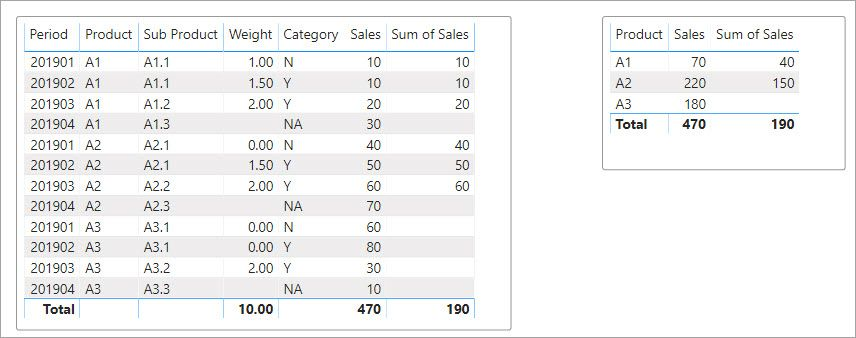cancel
Showing results for
Did you mean:Anonymous
Not applicable

## sum by comparing 3 columns

Hi All,

i have issue, where in i have to find the sum of sales by comparing other columns in the sames table. Please find the below sample

Table:

Product     Sub product      Sales   Weight

A1                 A1.1                       10          1

A1                A1.2                        20          2

A1                A1.3                        30

A2                A2.1                        40          4

A2                A2.2                        50          5

A3                A3.1                        60          6

A3                A3.2                        70          7

Expected Result:

Product     Sales

A1

A2                 90

A3                  130

If there is Weight available for every "sub product" then only i have to get the sum of sales for that "Product" else it should appear as 0 or "Blank".

Hope this all make sense and many thanks for your help !

Regards,

Vinay

2 ACCEPTED SOLUTIONSSuper User

Hello @Anonymous

Give this a try.

```Measure =
VAR _Blanks = CALCULATETABLE ( VALUES ( YourTable[Product] ), ALL ( YourTable ), ISBLANK ( YourTable[Weight] ) )
RETURN
CALCULATE(
SUM ( YourTable[Sales] ),
KEEPFILTERS ( NOT YourTable[Product] IN ( _Blanks ) )
)```Super User

@Anonymous

This gives me the expected result on the sample data and correct totaling as well.  Give it a try:

``````Sum of Sales =
SUMX (
VALUES ( 'Table'[Product] ),
CALCULATE (
VAR _Blanks =
CALCULATETABLE (
FILTER (
CALCULATETABLE (
GROUPBY ( 'Table', 'Table'[Product], 'Table'[Sub Product] ),
'Table'[Category] <> "NA"
),
"TheWeight", CALCULATE ( SUM ( 'Table'[Weight] ) )
),
[TheWeight] = 0
),
ALLEXCEPT ( 'Table', 'Table'[Product] ) )
RETURN
CALCULATE (
SUM ( 'Table'[Sales] ),
'Table'[Category] <> "NA",
FILTER ( 'Table', COUNTROWS ( _Blanks ) = BLANK () ) ) ) )``````5 REPLIES 5Super User

Hello @Anonymous

Give this a try.

```Measure =
VAR _Blanks = CALCULATETABLE ( VALUES ( YourTable[Product] ), ALL ( YourTable ), ISBLANK ( YourTable[Weight] ) )
RETURN
CALCULATE(
SUM ( YourTable[Sales] ),
KEEPFILTERS ( NOT YourTable[Product] IN ( _Blanks ) )
)```Anonymous
Not applicable

Thanks  !   i got my result.

Regards,

vinayAnonymous
Not applicable

Really Sorry, i'm coming back to you with a old problem with slight different case.

your Previous DAX worked fine, i'm facing problem to modify that with the below cases.

I'm stuck with same issue again with some complexity, please let me know, how can it be done.

Table:

Period   Product     Sub product      Sales   Weight      Category

201901      A1                A1.1                     10          1                N

201902      A1                A1.1                     10          1.5            Y

201903     A1                 A1.2                     20          2                Y

201904     A1                A1.3                      30                          NA

201901      A2               A2.1                      40         0                N

201902      A2                A2.1                     50          1.5          Y

201903     A2                A2.2                      60          2               Y

201904     A2                A2.3                      70                          NA

201901      A3               A3.1                      60         0                N

201902      A3                A3.1                     80          0               Y

201903     A3                A3.2                      30          2               Y

201904     A3                A3.3                      10                          NA

Here is in this scenario, weight is not calculated for "NA" Category.

Case  1: For Product "A1", i have positive weight for all the Sub Produts, so i have to calculate sum of sales for Product A1 = 40(10+10+20) , Excluding sales of NA(30)

Case 2 : For Product "A2", i have positive sales for all the sub category(here we have to consider sum  of weight of sub prorduct A1.1, which is positive(0+1.5)  ) then i  have calculate sum of sales , which is 150 (40+50+60)

Case 3 : For Product "A3", there is no positive weight for A3.1 Sub Product, so we should not calculate sum sales for this Product i.e A3 = blank

Result Table :

Product     Sum of sales

A1                40

A2               150

A3

How can this be done using DAX.  Please suggest

Sorry to trouble you with old problem again

VinaySuper User

@Anonymous

This gives me the expected result on the sample data and correct totaling as well.  Give it a try:

``````Sum of Sales =
SUMX (
VALUES ( 'Table'[Product] ),
CALCULATE (
VAR _Blanks =
CALCULATETABLE (
FILTER (
CALCULATETABLE (
GROUPBY ( 'Table', 'Table'[Product], 'Table'[Sub Product] ),
'Table'[Category] <> "NA"
),
"TheWeight", CALCULATE ( SUM ( 'Table'[Weight] ) )
),
[TheWeight] = 0
),
ALLEXCEPT ( 'Table', 'Table'[Product] ) )
RETURN
CALCULATE (
SUM ( 'Table'[Sales] ),
'Table'[Category] <> "NA",
FILTER ( 'Table', COUNTROWS ( _Blanks ) = BLANK () ) ) ) )``````Anonymous
Not applicable

@jdbuchanan71

Thanks a lot !. that was really helpful  🙂

Regards,

Vinay